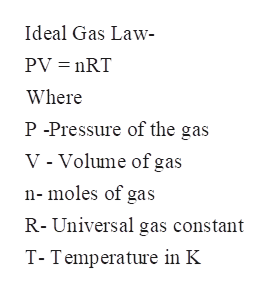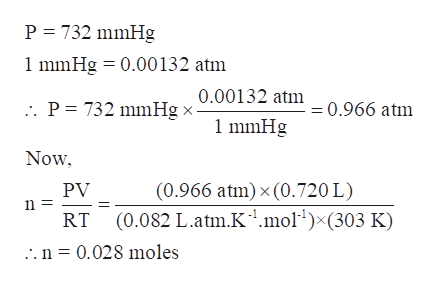# The hydrogen gas formed in a chemical reaction is collected over water at 30.0 ∘C at a total pressure of 732 mmHg .If the total volume of gas collected is 720 mL , what mass of hydrogen gas is collected?

Question
108 views

The hydrogen gas formed in a chemical reaction is collected over water at 30.0 ∘C at a total pressure of 732 mmHg .

If the total volume of gas collected is 720 mL , what mass of hydrogen gas is collected?

check_circle

Step 1

The hydrogen gas formed in a chemical reaction is collected over water at 30.0 ∘C at a total pressure of 732 mmHg. If the total volume of gas collected is 720 mL, the mass of hydrogen gas collected is to be calculated.

Step 2

Assuming the gas to be an ideal gas, the ideal gas law can be used to determine the moles of hydrogen gas collected.   From the moles of gas, mass of gas collected can be calculated.help_outlineImage TranscriptioncloseIdeal Gas Law PV nRT Where P -Pressure of the gas V - Volume ofgas n-moles of gas R-Universal gas constant T-Temperature in K fullscreen
Step 3

Determine the moles of gas collected:

P = 732 mmHg

V = 720 mL = 0.720 L

R = 0.082 L.atm.mol-1.K...help_outlineImage TranscriptioncloseP 732 mmHg 1 mmHg 0.00132 atm 0.00132 atm . P = 732 mmHg x- =0.966 atm 1 mmHg Now PV in (0.966 atm) x (0.720 L) (0.082 L.atm.K1mol1)x(303 K) RT .n = 0.028 moles fullscreen

### Want to see the full answer?

See Solution

#### Want to see this answer and more?

Solutions are written by subject experts who are available 24/7. Questions are typically answered within 1 hour.*

See Solution
*Response times may vary by subject and question.
Tagged in

### Gas laws